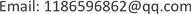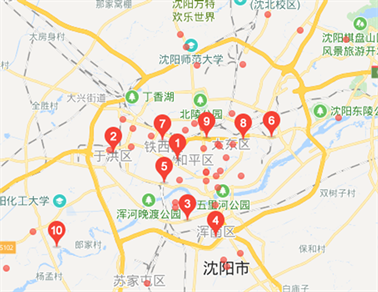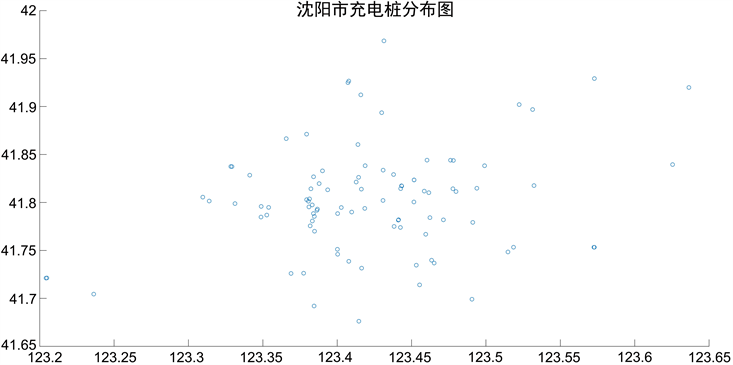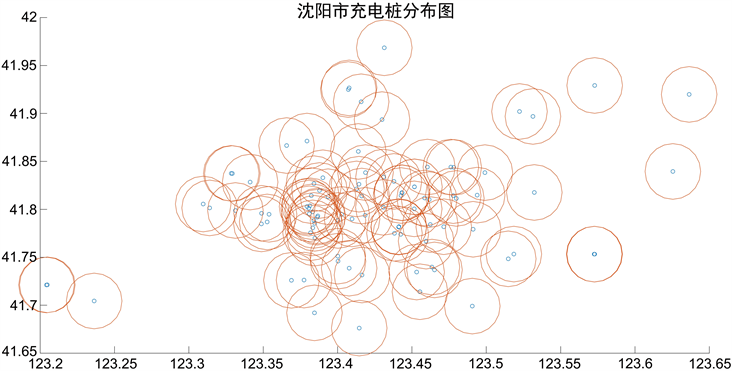﻿ 纯电动汽车的推广问题 Promotion of Pure Electric Vehicles

Vol. 08  No. 06 ( 2019 ), Article ID: 30884 , 7 pages
10.12677/AAM.2019.86131

Promotion of Pure Electric Vehicles

Xiangxin Song, Yu Liu, Zhen Zhuang, Manlong Chen

School of Science, Shenyang Aerospace University, Shenyang LiaoningReceived: Jun. 1st, 2019; accepted: Jun. 17th, 2019; published: Jun. 24th, 2019ABSTRACT

Pure electric vehicles are the representatives of new energy vehicles. Today, the country pays more and more attention to the construction of ecological environment, and its clean and pollution-free environmental protection advantages are gradually reflected. As the central city of the country, Shenyang’s sustainable development is bound to be the future development direction. In this regard, pure electric vehicles will usher in new opportunities for rapid development. How to improve the charging guarantee of pure electric vehicles is an important way to quickly promote pure electric vehicles and increase the market share of pure electric vehicles. This paper analyzes the current situation of the current charging station in Shenyang, and draws the shortcomings of the current number of charging stations and the defects in distribution. We also give rationalization advice on the future development of Shenyang charging station.

Keywords:Pure Electric Vehicle, Shenyang, Charging Station, Promotion, Opinion1. 纯电动汽车推广的必要性

2. 充电桩的数量情况。

$\left\{\begin{array}{l}N=\underset{i=1}{\overset{96}{\sum }}\frac{{T}_{1}}{{t}_{i}+\Delta t}\ast {n}_{i}+\underset{i=1}{\overset{96}{\sum }}\text{ }{\sigma }_{i}\ast {n}_{i}\\ {T}_{1}\in \left[8时,23时\right]\\ {T}_{2}\in \left[23时,次日8时\right]\end{array}$

$M=\beta \ast m$

$\beta$ 为一天中电动汽车需要充电的比例数，m为电动汽车的保有量。

3. 充电桩的分布情况Figure 1. Distribution of charging stations in Shenyang city

1) 充电站辐射半径

${\stackrel{¯}{r}}_{y}=\sqrt{\frac{S}{{n}_{y}}}$

${\stackrel{¯}{r}}_{d}=\theta \ast {\stackrel{¯}{r}}_{y}$

$\left\{\begin{array}{l}\text{A}区域=\left\{和平区,\text{\hspace{0.17em}}沈河区,\text{\hspace{0.17em}}大东区,\text{\hspace{0.17em}}皇姑区,\text{\hspace{0.17em}}铁西区\right\}\\ \text{B}区域=\left\{沈北新区,\text{\hspace{0.17em}}浑南区,\text{\hspace{0.17em}}于洪区,\text{\hspace{0.17em}}苏家屯区\right\}\end{array}$

$\left\{\begin{array}{l}{\stackrel{¯}{r}}_{dc}={\stackrel{¯}{r}}_{d}\ast \epsilon \text{\hspace{0.17em}}\text{\hspace{0.17em}}\text{\hspace{0.17em}}\text{\hspace{0.17em}}\text{\hspace{0.17em}}\text{A}区域\\ {\stackrel{¯}{r}}_{ds}={\stackrel{¯}{r}}_{d}\ast \delta \text{\hspace{0.17em}}\text{\hspace{0.17em}}\text{\hspace{0.17em}}\text{\hspace{0.17em}}\text{\hspace{0.17em}}\text{B}区域\end{array}$

2) 充电站的数量

$\left\{\begin{array}{l}{N}_{d}={n}_{dA}+{n}_{dB}=\frac{{S}_{A}}{{\stackrel{¯}{r}}_{dc}^{2}}+\frac{{S}_{B}}{{\stackrel{¯}{r}}_{ds}^{2}}\\ S={S}_{A}+{S}_{B}\\ {N}_{dz}={N}_{d}\ast \underset{i=1}{\overset{n}{\sum }}\text{ }{n}_{i}\end{array}$

3) 纯电动汽车的服务数量

$\left\{\begin{array}{l}{N}_{ct}=\underset{i=1}{\overset{n}{\sum }}\frac{{T}_{1}}{{t}_{i}+\Delta t}\ast {n}_{i}+\underset{i=1}{\overset{n}{\sum }}\frac{{T}_{2}}{{{t}^{\prime }}_{i}}\ast {n}_{i}\\ {N}_{c}={N}_{ct}\ast \gamma \\ {T}_{1}\in \left[8时,23时\right]\\ {T}_{2}\in \left[23时,次日8时\right]\end{array}$

4) 充电站的分布与辐射Figure 2. Distribution map of urban charging stations in ShenyangFigure 3. Radiation diagram of the charging station

4. 关于沈阳市纯电动汽车推广建议

1) 各车企要加大对研发环节的支持力度，提高纯电动汽车在技术领域的创新  。针对沈阳的冬天较为寒冷气候情况，重点对电池的快充技术、轻量化技术、储能技术、释能技术的研发和突破，使纯电动汽车不会因为气候寒冷而使运行里程大幅度下降，提高电动汽车的耐用度。同时整合汽车制造能力及合成技术，完善技术标准，提高纯电动汽车的安全性能和舒适度，制造出让消费者满意的纯电动汽车。

2) 积极向纯电动汽车推广较好的，重点是向与沈阳市城市地位相当城市的学习，如苏州，南京，青岛，杭州，合肥，大连等城市的学习，借鉴相关的经验，并结合沈阳的实际情况进行因地制宜的推广，不可盲目的照搬照抄。同时，政府部门要出台相关的鼓励性措施，在政策和财政上加大对纯电动汽车的支持力度，形成良好的的政策性导向。同时在公共交通领域，政府部门起到带头作用，引进纯电动汽车逐渐淘汰传统的燃油汽车，不仅能够让公众体会到纯电动汽车的优势，还可做到城市交通的升级。

3) 加大对纯电动汽车配套设施的建设，能够做到电动汽车的配套服务。根据沈阳市政府发布的《电动汽车充电基础设施建设总体方案(2016~2020年)》及实施细则，要求新建及改扩建住宅要100%配建或预留汽车充电桩，办公类建筑不低于20%，商业建筑及公共停车场不低于15%，这就为充电桩在沈阳市的普及创造出了良好的条件，在今后充电桩可能会在沈阳市的各个角落中出现，这就为纯电动汽车的推广创造了良好的条件。在建设充电桩时，要结合数量与分布两个方面进行，不能彼此间相互忽略。

4) 加强对群众可持续发展理念及环保意识的宣传，提高公众的环保意识，充分发挥纯电动汽车的环保性能。在今后沈阳市人民政府和下属的各级政府，各职能宣传机构要加强对环保知识的宣传，积极响应国家对生态文明建设的号召，不仅能够提高公民的节能环保意识，还能够更好的促进纯电动汽车的推广。

5) 推进传统加油站营销结构的升级。随着电动汽车的不断推广，传统的燃油汽车将会逐步被淘汰，这无疑会对加油站的业务产生较大的冲击。对此加油站可以考虑利用自己区位优势，建设充电桩等配套设施，增加相关的充电业务，形成油、电二位一体的多元营销模式  。

5. 结论

Promotion of Pure Electric Vehicles[J]. 应用数学进展, 2019, 08(06): 1133-1139. https://doi.org/10.12677/AAM.2019.86131

1. 1. 沈阳市人民政府网. http://www.shenyang.gov.cn/index.html

2. 2. 张洁, 裴梓翔. 国内纯电动汽车发展策略研究[J]. 能源与环境, 2017(5): 11-12.

3. 3. 苏映彰. 基于因子分析的电动汽车政策推广研究[J]. 现代商业, 2017(21): 36-38.

4. 4. 高悦. 新能源汽车在城市交通中的应用与推广[J]. 产品科技与论坛, 2018, 17(5): 59-60.

5. 5. 李博旭. 我国纯电动汽车发展面临的挑战及路径选择[J]. 汽车与配件, 2018(5): 48-50.

6. 6. 罗艳托, 汤湘华. 全球电动车发展趋势及未来研究[J]. 国际石油经济, 2018, 26(7): 58-64.